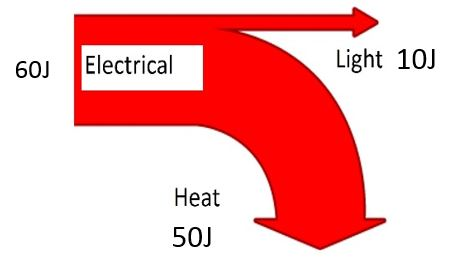# Energy Transfer

## Energy Transfer

Energy exists in different forms (stores) and is transferred from one store to another.

Examples of energy stores:

1. Light
2. Sound
3. Heat
4. Chemical
5. Electrical
6. Kinetic (movement)
7. Gravitational Potential (Stored by objects at a height)
8. Elastic Potential (Stored by elastic materials)

Energy Transfer

Devices transfer energy from one store (form) into another.

1. A light bulb converts electrical energy into light
2. A loudspeaker converts electrical energy into sound

## Sankey Diagrams

A Sankey diagram shows the energy transfers for a particular device.

1. The energy supplied is shown at the start of the arrow
2. The useful energy is shown by the arrow pointing straight ahead
3. ‘Wasted’ energy is shown by the arrow curving away from the useful energy

The size of the arrows represents the amount of energy.Example Sankey Diagrams:

## Elastic Potential Energy

Elastic Potential Energy (EPE) is stored in elastic materials when they are stretched or squashed. The EPE causes them to move back to their original shape when released.

For example:1. Energy is used to stretch a catapult
2. EPE is stored in the the catapult while it is stretched
3. EPE released (transformed into Kinetic Energy) when the catapult is released, causing the object to move forward

Elastic Potential Energy can be calculated using the following equation (you do NOT need to memorise this equation)

EPE = (ke2)÷2

__EPE __= Elastic Potential Energy (J)

K = spring constant (N/m)

e = extension (m)

Worked Example 1

Calculate the EPE stored in a spring with spring constant 250N/m when it is stretched to extend by 0.2m

EPE=ke2÷2

EPE=250 x 0.22÷2

EPE= 5J

Worked Example 2

If a spring with spring constant 300N/m has 20J of EPE, how much has it extended?

e= √(2EPE÷ k)

e= √(2x20÷ 300)

e= 0.37m

## Kinetic Energy

Kinetic energy is the energy possessed by a moving object. It depends on the velocity (speed) of the object and the mass of the object.

Kinetic Energy can be calculated using this equation: (you DO need to memorise this equation)

KE = (mv2)÷2

KE = Kinetic Energy (J)

m = mass (kg)

v = velocity (m/s)

Rearranging this equation to find velocity can be a bit tricky!

V = √(2KE ÷ m)

Worked Example

Calculate the kinetic energy of a man of mass 80kg running at a speed of 7m/s.

KE = (mv2)÷2

KE = (80 x 72) ÷2

KE = 1960J

## Gravitational Potential Energy

Gravitational Potential energy is the energy stored by an object due to the gravitational field of the Earth (or other planet). As you lift an object higher above the Earth, it gains gravitational potential energy. If the object falls back down again, it loses gravitational potential energy.

Gravitational Potential Energy can be calculated using this equation: (you DO need to memorise this equation)

GPE = mgh

GPE = gravitational potential energy

m = mass (kg)

__g __= gravitational field strength (9.81 N/kg on the Earth)

h = height (m)

__OR __(because W=mg), this equation can be used:

GPE = Wh

W = weight (N)

Worked Example

Calculate the gravitational potential energy gained by a pupil of mass 50kg who walks up 3 flights of stairs to a height of 15m. (gravitational field strength = 9.81 N/kg)

GPE = mgh

GPE = 50 x 9.81 x 15

GPE = 7358J

## Dissipation of Energy

1. Friction is a force exerted on an object sliding across another object
2. Friction transfers energy as heat
3. Heat energy is transferred to the surroundings which become slightly warmer
4. As energy dissipates (spreads out) it gets less and less useful
5. This dissipated energy is commonly referred to as ‘wasted’ energy

Watch the following video to see an example of conservation and dissipation of energy in action:

In this video, the bowling ball does not hit the person’s face because:

1. GPE is converted into KE as the ball travels downwards
2. KE is converted back into GPE as the ball travels up the other side
3. As the ball travels through the air it encounters air resistance
4. This means some energy is __dissipated __as heat in the air
5. As some of the energy has been dissipated, the ball will not have as much GPE at the end of its journey as it did at the start
6. Therefore the ball will not travel as high as its starting point
What is the energy input for an acoustic guitar?
kinetic
What is the increase in Gravitational Potential Energy when an object of weight 120N is lifted to a height of 5m?
600
How much kinetic energy does a man of mass 75kg have if he is walking at a speed of 2m/s?
150
At the top of the track, a rollercoaster has 1500J of GPE. Ignoring friction, how much Kinetic Energy would the rollercoaster have at the bottom of the track?
1500